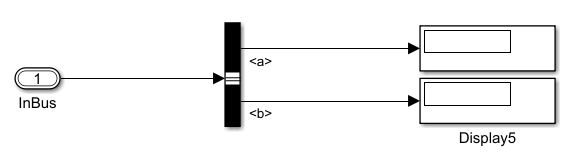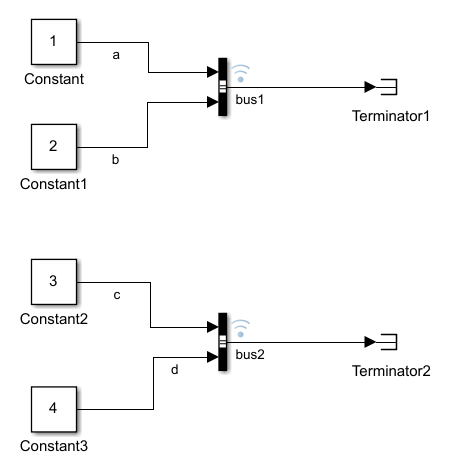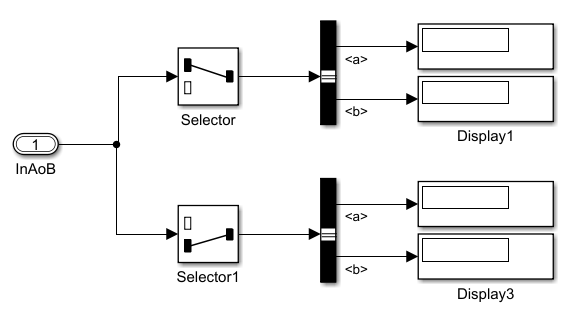Create structure of `timeseries` data to load as simulation input for bus

## Syntax

``tsStruct = Simulink.SimulationData.createStructOfTimeseries(busObj,tsStructIn)``
``tsStruct = Simulink.SimulationData.createStructOfTimeseries(busObj,tsCellArray)``
``tsStructArray = Simulink.SimulationData.createStructOfTimeseries(busObj,tsCellArray,dims)``
``tsStruct = Simulink.SimulationData.createStructOfTimeseries(tsArray)``

## Description

example

````tsStruct = Simulink.SimulationData.createStructOfTimeseries(busObj,tsStructIn)` creates a structure with attributes that match those specified by the `Simulink.Bus` object, `busObj`, and data specified by the structure of `timeseries` objects, `tsStructIn`. When the names in the input structure do not match the names specified by the bus object, the function renames the fields in the output structure to match the bus object specification. When other attributes such as data type and complexity do not match, the function returns an error. You can use this syntax to create a simulation input that fully or partially specifies the data for a bus. You can also use this syntax to rename the structure elements to match the names in the `Simulink.Bus` object.```

example

````tsStruct = Simulink.SimulationData.createStructOfTimeseries(busObj,tsCellArray)` creates a structure of `timeseries` objects with attributes that match those specified by the `Simulink.Bus` object, `busObj`, and data specified by the cell array of `timeseries` objects, `tsCellArray`. When the names in the input structure do not match the names specified by the bus object, the function renames the fields in the output structure to match the bus object specification. When other attributes such as data type and complexity do not match, the function returns an error. You can use this syntax to create simulation input that fully or partially specifies the data for a bus using a flat list of `timeseries` objects. The function maps the `timeseries` objects to the hierarchy specified by the `Simulink.Bus` object using a depth-first search.```

example

````tsStructArray = Simulink.SimulationData.createStructOfTimeseries(busObj,tsCellArray,dims)` creates an array of `timeseries` structures where the attributes of each structure match those defined by the `Simulink.Bus` object, `busObj` with the data specified by the cell array of `timeseries` objects, `tsCellArray`. The input `dims` specifies the dimensions of the array.```

example

````tsStruct = Simulink.SimulationData.createStructOfTimeseries(tsArray)` creates a structure of `timeseries` objects from the bus data stored in the `Simulink.TsArray` object, `tsArray`. In versions before R2016a, signal logging creates `Simulink.TsArray` objects to store logged bus data. Use this syntax when you want to use bus data logged in a release before R2016a using `ModelDataLogs` format as simulation input.```

## Examples

collapse all

You can use the `Simulink.Simulationdata.createStructOfTimeseries` function to create structures of `timeseries` objects to use as simulation input for bus signals. This example shows you how to create a structure of timeseries to load into a model using timeseries data logged from a different simulation.

Create a Structure of Timeseries

Open the `ex_log_structtimeseries` model.

```open_system('ex_log_structtimeseries') ```The model uses Constant blocks and Bus Creator blocks to build two buses, `bus1` and `bus2`, with signals `a`, `b`, `c`, and `d`. The model uses signal logging to log the bus data. Create bus data by simulating the model.

```out = sim('ex_log_structtimeseries'); ```

View the data in the logging variable `logsout`. Signal logging creates a `Simulink.SimulationData.Dataset` object with `Simulink.SimulationData.Signal` objects as elements.

```logsout = out.logsout ```
```logsout = Simulink.SimulationData.Dataset 'logsout' with 2 elements Name BlockPath ____ ____________________________________ 1 [1x1 Signal] bus1 ex_log_structtimeseries/Bus Creator 2 [1x1 Signal] bus2 ex_log_structtimeseries/Bus Creator1 - Use braces { } to access, modify, or add elements using index. ```

You can use the `get` function to select the `Simulink.SimulationData.Signal` object for `bus2`. The bus data is in the `Values` property of the `Simulink.SimulationData.Signal` object. The data representing `bus2` is logged in a structure containing `timeseries` objects named `c` and `d`.

```logsout.get(2).Values ```
```ans = struct with fields: c: [1x1 timeseries] d: [1x1 timeseries] ```

Open the `ex_load_structtimeseries` model, which uses the logged simulation data as input.

```open_system('ex_load_structtimeseries') ```The model uses the `InBus` Inport block to load input bus data. A Bus Selector block chooses signals from the bus to display on Display blocks.

Double-click the `InBus` block and check its Data type on the Signal Attributes tab of the dialog. The data type is specified by a `Simulink.Bus` object called `bus`.Close the dialog and open the Model Explorer. On the Callbacks tab, you can see the model uses its `PreLoadFcn` to define the `Simulink.Bus` object that defines the data type for the Inport block.

Open the Configuration Parameters and view the specification for the Input parameter on the Data Import/Export pane. The model uses the variable `inputBus` for its Input.

Create Simulation Input from the Structure of `timeseries` Data

To load the data logged for `bus1`, you only need to assign the structure data to the Input variable for the model.

```inputBus = logsout.get(1).Values ```
```inputBus = struct with fields: a: [1x1 timeseries] b: [1x1 timeseries] ```

When you simulate the model, the Display blocks show the values `1` and `2` logged in `bus1` and loaded into the model.

To load the data logged for `bus2`, you need to use the `Simulink.Bus` object that defines the Inport block data type and `Simulink.SimulationData.createStructOfTimeseries` to create a structure of `timeseries` with names specified by the `Simulink.Bus` object.

```inputBus = Simulink.SimulationData.createStructOfTimeseries('bus',... logsout.get(2).Values) ```
```inputBus = struct with fields: a: [1x1 timeseries] b: [1x1 timeseries] ```

When you simulate the model, the Display blocks show the values `3` and `4` logged in `bus2` and loaded into the model.

This example shows how to use the `Simulink.SimulationData.createStructOfTimeseries` function to create partially specified simulation input for a bus. This example logs data from `ex_log_structtimeseries` and then loads that data into `ex_load_structtimeseries`.

Create `timeseries` Data

First, open and simulate the `ex_log_structtimeseries` model. The model logs two bus signals, `bus1` and `bus2`, created using Constant blocks and Bus Creator blocks. Access the `logsout` `Dataset` in the `Simulink.SimulationOutput` object, `out`.

```open_system('ex_log_structtimeseries') out = sim('ex_log_structtimeseries'); logsout = out.logsout; ```You can use a structure of `timeseries` data or a cell array of `timeseries` data to partially specify simulation input for a bus.

Partially Specify Bus Data with a Structure of `timeseries` Data

Open the model `ex_load_structtimeseries` that will load some of the data you logged in the previous section.

```open_system('ex_load_structtimeseries') ```Use the `get` function to access the structure of `timeseries` data logged for `bus1`.

```bus1 = logsout.get(1).Values; ```

Then, replace the `b` data with `[]`.

```bus1.b = []; ```

The `ex_load_structtimeseries` model uses the variable `inputBus` as its Input. The `Simulink.Bus` object, `bus`, that defines the data type for the Inport block is defined in the `PreLoadFcn` callback for the `ex_load_structtimeseries` model. Because the signal names in `bus1` match the `Simulink.Bus` object specification for the Inport block in the `ex_load_structtimeseries` model, you can use the logged structure without modification. To load the data for `bus1`, assign `bus1` to the variable `inputBus`.

```inputBus = bus1; ```

Simulate the model. The Display blocks show the logged value `1` for `a` and `0` for `b`. The simulation uses ground values when you do not specify data for the signal.

```loadOut = sim('ex_load_structtimeseries'); ```

Now, load the data logged for `bus2`. The signal names in `bus2` do not match the `Simulink.Bus` object specification for the Inport block in the `ex_load_structtimeseries` model. Modify the data in the structure to partially specify input data for the bus. Then, use the `Simulink.SimulationData.createStructOfTimeseries` function to change the names in the structure to match the bus specification.

```bus2 = logsout.get(2).Values; bus2.d = []; inputBus = bus2; inputBus = Simulink.SimulationData.createStructOfTimeseries('bus',inputBus); ```

Simulate the model. The Display blocks show the logged value `3` for `a` and `0` for `b`.

```loadOut = sim('ex_load_structtimeseries'); ```

Partially Specify Bus Data with a Cell Array of Timeseries Data

When you have `timeseries` data, you can use `Simulink.SimulationData.createStructOfTimeseries` to partially specify simulation input for a bus using a cell array of the `timeseries` data. Load the `timeseries` data for signal `d` in `bus2` as part of a partial bus specification for the Inport block in the `ex_load_structtimeseries` model. The `PreLoadFcn` callback for the `ex_load_structtimeseries` model defines the `Simulink.Bus` object, `bus`, that defines the data type for the Inport block.

```d = logsout.get(2).Values.d; inputBus = Simulink.SimulationData.createStructOfTimeseries('bus',... {d,[]}); ```

Simulate the model. The Display block for signal `a` in the `ex_load_structtimeseries` model shows the data logged in signal `d` from the `ex_log_structtimeseries` model. The Display block for signal `b` shows `0`.

```loadOut = sim('ex_load_structtimeseries'); ```

This example shows how to use the `Simulink.SimulationData.createStructOfTimeseries` function to generate simulation input for an array of buses. You create `timeseries` data by simulating one model. Then, you create an input structure using the logged data to load into an array of buses in another model.

Create `timeseries` Data

To start, open the `ex_log_structtimeseries` model.

```open_system('ex_log_structtimeseries') ```The model creates two buses, `bus1` and `bus2`, using Constant blocks and Bus Creator blocks. The signals are named `a`, `b`, `c`, and `d`. Create logged bus data by simulating the model.

```out = sim('ex_log_structtimeseries'); ```

The output `out` contains a `Simulink.SimulationData.Dataset` object, `logsout`, with the logged data. You can access the `bus1` and `bus2` signals using the `get` function. The data for each signal is in the `Simulink.SimulationData.Signal` object `Values` parameter. You can access the bus elements using a dot followed by the signal name. `bus1` is the first signal in the `Dataset` object and contains signals `a` and `b`. `bus2` contains signals `c` and `d`.

```logsout = out.logsout; a = logsout.get(1).Values.a; b = logsout.get(1).Values.b; c = logsout.get(2).Values.c; d = logsout.get(2).Values.d; ```

Open the model `ex_structtimeseries_aob`, which uses an array of buses as input.

```open_system('ex_load_structtimeseries_aob') ```The model uses the `InAoB` Inport block to load simulation input. Selector blocks select a bus from the array of buses, and Bus Selector blocks select signals to show in the Display blocks.

Double-click the `InAoB` block and look at the Signal Attributes pane of the dialog. The Data type for the block is set to Bus with the type defined by the `Simulink.Bus` object, `bus`. The Port dimensions parameter is set to `[2 1]`.

You can see the definition for the `Simulink.Bus` object, `bus`, in the Callbacks tab in the Model Explorer. This model uses the `PreLoadFcn` to define the bus object.

Open the Model Configuration Parameters and look at the Input parameter. The model uses the variable `inputAoB` as input.

Create Array of Buses Simulation Input

Use `Simulink.SimulationData.createStructOfTimeseries` and the data logged in the first section to create a structure to load as simulation input for the array of buses. Specify the dimensions as `[2 1]` to match the dimensions of the `InAoB` block.

```inputAoB = Simulink.SimulationData.createStructOfTimeseries('bus',... {a,b,c,d},[2 1]); ```

When you simulate the model, the Display blocks show the data for signals `a`, `b`, `c`, and `d` logged from the `ex_log_structtimeseries` model. The array of buses contains two buses with signals `a` and `b`. `Simulink.SimulationData.createStructOfTimeseries` renamed signals `c` and `d` to match the `Simulink.Bus` specification used by the array of buses.

```inputAoB(2) ```
```ans = struct with fields: a: [1x1 timeseries] b: [1x1 timeseries] ```

Simulate the model. The display blocks show the logged values.

```aob_out = sim('ex_load_structtimeseries_aob'); ```

In releases before R2016a, when you log simulation data using `ModelDataLogs` format, bus data is stored as a `Simulink.TSArray` object. You cannot log data using `ModelDataLogs` format using a release after R2016a. In this example, the logged data, `logsout`, was logged in `ModelDataLogs` format using a release before R2016a. The variable `logsout` contains data for a single bus, `bus1`.

```logsout ```
```logsout = Simulink.ModelDataLogs (log_modeldatalogs): Name Elements Simulink Class bus1 2 TsArray```

To load the logged data as simulation input for a bus, create a structure of `timeseries` objects from the data in `bus1`.

```struct_of_ts = ... Simulink.SimulationData.createStructOfTimeseries(logsout.bus1)```
```struct_of_ts = const1_sig: [1x1 timeseries] const2_sig: [1x1 timeseries]```

## Input Arguments

collapse all

Name of the `Simulink.Bus` object that specifies the attributes for the data in the output structure of `timeseries` objects. When you want to load the structure of `timeseries` objects as simulation input for a bus, the `busObj` is the bus that defines the data type for the root-level Inport block.

`Simulink.SimulationData.createStructOfTimeseries` validates the input `timeseries` attributes including data type and complexity against the `Simulink.Bus` object specification. When element names do not match between the `Simulink.Bus` specification and the input `timeseries` data, `Simulink.SimulationData.createStructOfTimeseries` renames the `timeseries` data to match the bus specification. When other attributes do not match, the function returns an error.

Example: `'MyInputBus'`

Structure of `timeseries` data for use in creating the output structure of `timeseries` objects according to the `Simulink.Bus` object. The structure must have the same hierarchy as the `Simulink.Bus` object.

To partially specify data for a bus, use `[]` in the place of the bus element you want to use ground values.

Cell array of `timeseries` objects specifying the data for the output structure of `timeseries` objects.

To partially specify data for a bus, use `[]` in the place of the bus element you want to use ground values.

The `Simulink.SimulationData.createStructOfTimeseries` function maps the `timeseries` elements of the cell array to the hierarchy specified by the `Simulink.Bus` object using a depth-first search.

Example: `{ts1,ts2,ts3}`

Example: `{ts1,[],ts3}`

#### Dependencies

When you specify the `dims` argument, the number of cells in the cell array must match the number of individual signal elements in the `Simulink.Bus` object multiplied by the product of the specified dimensions.

Dimensions for the array of `timeseries` structures, specified as a vector.

When you specify the dimensions as a scalar, `n`, the function creates a `1`-by-`n` array.

Example: `[2,1]`

#### Dependencies

When you specify the `dims` argument, the number of cells in the cell array must match the number of individual signal elements in the `Simulink.Bus` object multiplied by the product of the specified dimensions.

Data Types: `double`

`Simulink.TsArray` object.

In versions prior to R2016a, signal logging creates `Simulink.TsArray` objects to store logged bus data. Use this syntax when you want to use data logged using `ModelDataLogs` format in a version before R2016a to create simulation input for a bus.

Example: `myTsArrayObj`

## Output Arguments

collapse all

Structure of `timeseries` objects with attributes specified by the `Simulink.TsArray` or `Simulink.Bus` input. You can load the structure of `timeseries` objects as simulation input for a bus.

Array of structures of `timeseries` objects with dimensions specified by the `dims` input.

## Version History

Introduced in R2013a